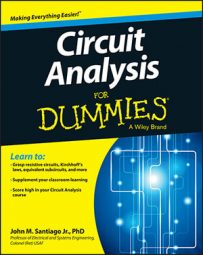##### Circuit Analysis For DummiesCircuit analysis is a tricky subject, and it’s easy to make certain mistakes, especially when you’re first starting out. You can reduce your odds of making these common mistakes by reviewing the following list.

## Failing to label voltage polarities and current directions

When you analyze any circuit, the first step is to properly label the voltage polarities and current direction for each device in the circuit. Circuit labels serve as reference marks for what’s happening in the circuit.

## Making common math errors in circuit design

Simple arithmetic and algebraic errors can cost you when designing circuits. If your calculation is off by one decimal place, your circuit won’t work as designed. Basic trigonometric errors are problematic too, because if you don’t get the trig right, you’ll mess up important calculations involving imaginary and complex numbers.

And when you’re working with first- and second-order circuits, you need to avoid calculus errors, too. Always pay attention to your calculations and double-check your math.

## Making incorrect assumptions about open and short circuits

You can’t assume the voltage across an open circuit is zero just because the current through an open circuit is zero. Likewise, you can’t assume the current through a short circuit is zero just because the voltage across the short circuit is zero.

Instead, remember that an open circuit has infinite resistance, having any voltage value across the open circuit but no current through it. The short circuit is like a piece of wire having zero resistance, so any amount of current can flow through it.

## Forgetting that the voltage across a current source can be any value

Some students assume that the voltage across a constant current source is zero, but that’s not the case. The voltage across a current source can be any value.

Students who make this mistake often forget that in terms of resistance, a constant current source is a device having infinite resistance (like an open circuit). And an open circuit can have any voltage across it. In fact, using a constant current source with a value of zero is equivalent to replacing the current source with an open circuit.

## Misidentifying series and parallel device connections

Beginning circuit analysis students frequently struggle to identify the difference between series and parallel connections of devices. To avoid making this mistake, commit the following to memory:

• Series connections: For two devices to be connected in series, only those two devices can share a common junction point (or node). If three or more devices share a node, the devices aren’t in series. All devices connected in series share a common current.

• Parallel connections: Devices connected in parallel must share two common junction points (or nodes). All devices connected in parallel share a common voltage.

## Simplifying circuits incorrectly

Simplifying complex circuits can make your analysis easier (plus you can try different loads to meet circuit requirements). But if you mess up the simplification, you inevitably lose terminals, resulting in a circuit that isn’t equivalent.

Make sure you don’t lose the terminals of interest as you simplify the circuit. If you’re interested in a specific pair of terminals, one approach is to convert the circuit so that all elements are connected in parallel or all elements are connected in series at the pair of terminals.

## Applying transformed circuits incorrectly

When you do source transformation, remember that the two circuits are equivalent only at the terminals of interest. If you forget this, you can misinterpret the actual output value.

Verify your transformed circuit with other circuit analysis techniques derived from Kirchhoff’s voltage and current laws, such as source transformation and voltage and current divider techniques. Or you can simply apply Kirchhoff’s laws to check your results.

## Formulating node voltage equations incorrectly

The purpose of node-voltage analysis is to find the voltages across the devices in a circuit. A common mistake is attempting to write a nodal equation through a voltage source when none of its terminals are connected to ground. This approach isn’t valid because a basic nodal equation can’t be written through a voltage source. Instead, you must treat the voltage source for this special case as one node (known as a supernode).

Another common pitfall with node-voltage analysis concerns how students visualize a node in a circuit diagram as one specific point in a circuit. When a node has three or more branches, students tend to make multiple nodes out of a single node.

Verify your work in node-voltage analysis when you only have independent sources by looking at the symmetry of the matrix of conductances along the diagonal. If it’s not symmetric, then you did something incorrectly. Also remember that when you have dependent sources, you may or may not have symmetry along the matrix diagonal.

## Setting up mesh current equations incorrectly

You can’t form a mesh current equation when your mesh includes a current source, because the voltage across a current source can be any value. You need to avoid (or bypass) the current source so it’s not part of the circuit mesh equations. If the current source is part of two meshes, then you can form one supermesh to write the mesh current equation.

For consistency, make your mesh currents point in the same (clockwise) direction. You’ll end up with a matrix of resistances that’s symmetric, with positive elements along the main diagonal and negative off-diagonal elements. Better yet, if your resulting matrix has these characteristics, then you’ll know that you’ve likely formulated the mesh current equations correctly.